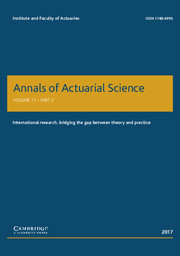Home
Hostname: page-component-888d5979f-g2njx Total loading time: 0.179 Render date: 2021-10-26T19:48:09.825Z Has data issue: true Feature Flags: { "shouldUseShareProductTool": true, "shouldUseHypothesis": true, "isUnsiloEnabled": true, "metricsAbstractViews": false, "figures": true, "newCiteModal": false, "newCitedByModal": true, "newEcommerce": true, "newUsageEvents": true }Annals of Actuarial Science

# Ruin problems in Markov-modulated risk models

Published online by Cambridge University Press:  30 May 2017

*
*Correspondence to: David C.M. Dickson, Department of Economics, Centre for Actuarial Studies, The University of Melbourne, Melbourne, VIC 3010, Australia. Tel: +61 3 8344 4727. Fax: +61 3 8344 6899. E-mail: dcmd@unimelb.edu.au

## Abstract

Chen et al. (2014), studied a discrete semi-Markov risk model that covers existing risk models such as the compound binomial model and the compound Markov binomial model. We consider their model and build numerical algorithms that provide approximations to the probability of ultimate ruin and the probability and severity of ruin in a continuous time two-state Markov-modulated risk model. We then study the finite time ruin probability for a discrete m-state model and show how we can approximate the density of the time of ruin in a continuous time Markov-modulated model with more than two states.

## Keywords

Type
Papers
Information
Annals of Actuarial Science , March 2018 , pp. 23 - 48
© Institute and Faculty of Actuaries 2017

## Access options

Get access to the full version of this content by using one of the access options below. (Log in options will check for institutional or personal access. Content may require purchase if you do not have access.)

## References

Albrecher, H. & Boxma, O.J. (2005). On the discounted penalty function in a Markov-dependent risk model. Insurance: Mathematics & Economics, 37, 650672.Google Scholar
Ammeter, H. (1948). A generalisation of the collective theory of risk in regard to fluctuating basic probabilities. Scandinavian Actuarial Journal 1948, 171198.CrossRefGoogle Scholar
Asmussen, S. (1989). Risk theory in a Markovian environment. Scandinavian Actuarial Journal 1989, 69100.CrossRefGoogle Scholar
Asmussen, S. & Albrecher, H. (2010). Ruin probabilities, 2nd edition. World Scientific, Singapore.CrossRefGoogle Scholar
Chen, M., Yuen, K.C. & Guo, J. (2014). Survival probabilities in a discrete semi-Markov risk model. Applied Mathematics and Computation, 232, 205215.CrossRefGoogle Scholar
De Vylder, F. & Goovaerts, M.J. (1988). Recursive calculation of finite-time ruin probabilities. Insurance: Mathematics & Economics, 7, 17.Google Scholar
Dickson, D.C.M., Egídio dos Reis, A.D. & Waters, H.R. (1995). Some stable algorithms in ruin theory and their applications. ASTIN Bulletin, 25, 153174.CrossRefGoogle Scholar
Dickson, D.C.M. & Waters, H.R. (1991). Recursive calculation of survival probabilities. ASTIN Bulletin, 21, 199221.CrossRefGoogle Scholar
Dickson, D.C.M. & Waters, H.R. (1992). The probability and severity of ruin in finite and infinite time. ASTIN Bulletin, 22, 177190.CrossRefGoogle Scholar
Dickson, D.C.M. & Waters, H.R. (2002). The distribution of the time to ruin in the classical risk model. ASTIN Bulletin, 32, 299313.CrossRefGoogle Scholar
Dufresne, F. (1988). Distributions stationnaires d’un système bonus-malus et probabilité de ruine. ASTIN Bulletin, 18, 3146.CrossRefGoogle Scholar
Grandell, J. (1991). Aspects of Risk Theory. Springer-Verlag, New York.CrossRefGoogle Scholar
Li, J., Dickson, D.C.M. & Li, S. (2014). Finite time ruin problems for the Markov-modulated risk model, Working paper, University of Melbourne, Melbourne.Google Scholar
Li, S. & Lu, Y. (2008). The decompositions of the discounted penalty functions and dividends-penalty identity in a Markov-modulated risk model. ASTIN Bulletin, 38, 5371.CrossRefGoogle Scholar
Lu, Y. (2006). On the severity of ruin in a Markov-modulated risk model. Scandinavian Actuarial Journal, 2006, 183202.CrossRefGoogle Scholar
Lu, Y. & Li, S. (2005). On the probability of ruin in a Markov-modulated risk model. Insurance: Mathematics & Economics, 37, 522532.Google Scholar
Ng, A.C.Y. & Yang, H. (2006). On the joint distribution of surplus before and after ruin under a Markovian regime switching model. Stochastic Processes and their Applications, 116, 224266.CrossRefGoogle Scholar
Panjer, H.H. (1981). Recursive evaluation of a family of compound distributions. ASTIN Bulletin, 12, 2126.CrossRefGoogle Scholar
Panjer, H.H. & Wang, S. (1993). On the stability of recursive formulas. ASTIN Bulletin, 23, 227258.CrossRefGoogle Scholar
Reinhard, J.M. (1984). On a class of semi-Markov risk models obtained as classical risk models in a Markovian environment. ASTIN Bulletin, 14, 2343.CrossRefGoogle Scholar
Reinhard, J.M. & Snoussi, M. (2002). The severity of ruin in a discrete semi-Markov risk model. Stochastic Models, 18, 85107.CrossRefGoogle Scholar
Snoussi, M. (2002). The severity of ruin in Markov-modulated risk models. Bulletin of the Swiss Association of Actuaries, 1, 3143.Google Scholar
1
Cited by

# Send article to Kindle

Note you can select to send to either the @free.kindle.com or @kindle.com variations. ‘@free.kindle.com’ emails are free but can only be sent to your device when it is connected to wi-fi. ‘@kindle.com’ emails can be delivered even when you are not connected to wi-fi, but note that service fees apply.

Find out more about the Kindle Personal Document Service.

Ruin problems in Markov-modulated risk models
Available formats
×

# Send article to Dropbox

To send this article to your Dropbox account, please select one or more formats and confirm that you agree to abide by our usage policies. If this is the first time you use this feature, you will be asked to authorise Cambridge Core to connect with your <service> account. Find out more about sending content to Dropbox.

Ruin problems in Markov-modulated risk models
Available formats
×

# Send article to Google Drive

To send this article to your Google Drive account, please select one or more formats and confirm that you agree to abide by our usage policies. If this is the first time you use this feature, you will be asked to authorise Cambridge Core to connect with your <service> account. Find out more about sending content to Google Drive.

Ruin problems in Markov-modulated risk models
Available formats
×
×